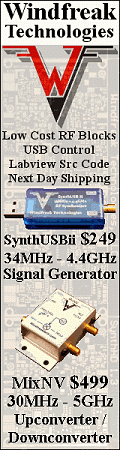Please Support RF Cafe by purchasing my  ridiculously low−priced products, all of which I created.

RF Workbench  (shareware)# Electronic Puzzle SquareJuly 1945 Radio-Craft

 July 1945 Radio-Craft[Table of Contents] Wax nostalgic about and learn from the history of early electronics. See articles from Radio-Craft, published 1929 - 1953. All copyrights are hereby acknowledged.

Due to the era in which this "Electronic Puzzle Square" appeared (1945), I made a couple edits to help prevent misinterpretation. For instance the "mfd." in question 5 is microfarad (μF) in today's units standard. Question 4 originally had an upper case "E," which was a typo since it should have been a lower case "e," as in the base of the natural logarithm. When working Q7, leave the input and output terminals open when calculating the equivalent resistance; it's not like doing a "Pi" to "Tee" attenuator conversion. Q11 originally had "logE" where it should have been just "e." Q9 is a piece of cake. Question 15 assumes you know the resistance per foot of #25 B&S (AWG) copper wire, which you can find here (hint: it's 32.4 Ω/1000 ft). Bon chance!

Here is another Electronic Puzzle Square from the Janaury 1945 issue.

Electronic Puzzle Square

By Lt. C. K. Johnson

Work this like a crossword puzzle. The answer to problem No. 1 goes in square No.1, etc. When completed, rows, columns and diagonals, etc., will total the number which is the base of common logarithms. Therefore the sum of the answers to problems 1, 2 and 3 subtracted from the total gives the answer to problem 4.(All problems correct to one decimal place.)

1. LogeN = ___ Log10N

2. What is R if I = 10 A and P = 320 W?

3. Red is ___ in the color code.

4. ex = ___ when x = 0.92

5. An inductance of 0.004 henrys is used with a condenser of what value in mfd. (μF) to obtain a resonant frequency of 1500 cps ?

6. Tangent 59.6° = ___.7. If we had a circuit as in Fig. 1 where each resistor was 10 ohms, and we wanted to convert it to a circuit as at Fig. 2 so that the resistance measured between A and B or measured between C and D, would be the same for each circuit, what resistors would we use in Fig. 2?8. What reading in milliamperes will be indicated by the meter?

9. The ratio of the circumference of a circle to its diameter is ___.10. What is the total resistance between A and B?

11. If e-x = 0.0743, what is x ?12. What would be the required voltage of battery to read 1.9 volts across R1, R2, and R3?

13. √π

14.  Base of the natural system of logarithms.

15.  At 77 °F, what would be the resistance of 64 feet of B and S gauge No. 25 bare copper wire?

16.  If an A.C. generator has an R.M.S. output of 2.4 volts, what is its peak output?

Here are other electronics-themed crossword puzzles from vintage electronics magazines (RF Cafe Crosswords here):Posted February 10, 2021